American Journal of Applied Mathematics
Volume 3, Issue 4, August 2015, Pages: 201-205

Application of Neural Network Model in an Epidemiological Study

Renhao Jin, Fang Yan, Jie Zhu

School of Information, Beijing Wuzi University, Beijing, China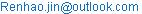(Renhao Jin)

Renhao Jin, Fang Yan, Jie Zhu. Application of Neural Network Model in an Epidemiological Study. American Journal of Applied Mathematics. Vol. 3, No. 4, 2015, pp. 201-205. doi: 10.11648/j.ajam.20150304.16

Abstract: This paper use the neural network model to an epidemiological study, i.e. bovine tuberculosis (bTB) occurrence in cattle herds, together with well-established risk factors in the area known as West Wicklow, in the east of Ireland. The binary target variable is whether the herd is in the restricted status, which is defined by whether any bTB reactor is detected in the herd. To estimate the parameters and prevent over-fitting in neural network model fitting, the observations are divided into three part of Training data set, Validation data set, and Test data set. By analysis on the lift charts on test data set, the fitted neural network model can be used to enhance practice efficiency.

Keywords: Neural Network Model, Bovine Tuberculosis, Spearman’s Rank Correlation, Lift Chart

Contents

1. Introduction

Neural network model is also regarded as artificial neural networks, and it is widely used in a lot of fields as a statistical learning model. The neural model is inspired by the biological neural net, and it is a predictive method with higher precision and larger computation costs comparing with logistic regression model and decision tree model. Neural network models are used to estimate weights and functions in the network depending on a large number of inputs and outputs. The flow chart of neural model are shown in Figure 1 and Figure 2. In the figure 1, the input and output are similar to the independent and dependent variable in regression models respectively. The hidden layer is the unique part of neural model, and it may have several hidden layers in a neural model. Each circle in the hidden layer is called a hidden layer node. In general, one hidden layer is adequate for the estimation precision. More hidden layers may increase the prediction precision but the computation cost increases exponentially.

Figure 2 is the detailed connection for all inputs, a hidden layer node and the output. As shown in Figure 1 and 2, the inputs are firstly weighted linear combined and then transferred to each hidden layer node. In each hidden layer node, a transfer function (often nonlinear function) is applied on the linear combination and then the results are passed to the output layer. Similar to the inputs, all the computation results from each hidden layer node are also linear combined and pass to the output. In the output node, an activation function is also applied to the linear combination from hidden layer nodes and then output the final results. Generally speaking, linear activation function is used for continuous target output, while nonlinear activation function is for discrete target variable.

The epidemiological study in this paper is based on aggregated bovine tuberculosis (bTB) data in cattle herds from 2005 to 2009, together with well-established risk factors in the area known as West Wicklow, in the east of Ireland. The bTB data is from the first author’s Ph.D thesis, and the other related part of the bTB study has been published in Veterinary Record (2013).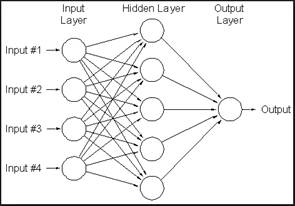Figure 1. The general flow chart of neural network model with input layer, hidden layer and output layer.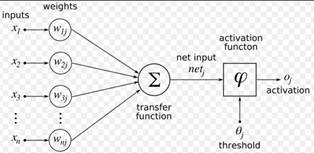Figure 2. The mathematical functions in the link of all inputs, a hidden layer node and output in a neural network model.

Bovine tuberculosis (bTB), caused by infection with Mycobacterium bovis, affects approximately 0.3% of cattle annually in Ireland, with 18,531 reactor cattle identified in 2011. This has major financial implications both for the farmer whose herd is restricted from trading and cattle slaughtered, and for the exchequer that compensates the farmer and implements measures to control the disease. Data for the bTB study were obtained from three sources: herd data from the national databases of bTB testing herd and animal history (Animal Health Computer System, AHCS); land usage from Herdfinder, a unique multi-layered purpose built spatial mapping system whereby farms shapes submitted by farmers to DAFM under the EU Single Farm Payment Scheme are recorded and weather data from Met Éireann, all for West Wicklow. Both AHCS and Herdfinder databases use the same herd ID number so that farm, geographic location and testing data may be linked. The spatial distribution of herds and rainfall stations, and the study areas is shown in Figure 3.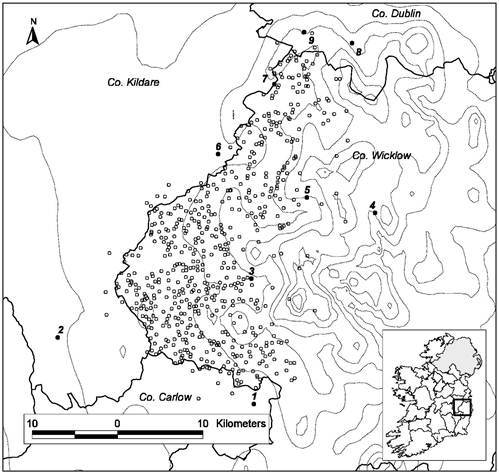Figure 3. The spatial distribution of herd observations and rainfall stations. Out of 14 rainfall stations, nine were the nearest to any one herd and they are shown in the map indexed by station numbers. The station names and numbers were: Hacketstown (1), Castledermot (2), Glen of Imaal (3), Glenmacnass (4), Glenbride Lodge (5), Ballymore (6), Blessington (7), Glenasmole D (8), and Brittas (9). The dashed lines in the map are contour lines with 100 metres intervals, i.e. joined points are equal to 100 metres height above the sea level.

2. Neural Network Modelling

A neural network model was built to predict bTB incidence in cattle herds based on the potential risk factors (explanatory variables) from 2005 to 2009. The herd target variable is binary, indicating whether any bTB reactor is detected in the herd, and the herd with target value 1 is with restriction status. So the response variable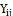is binary: restriction status of the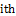herd in year(1=restricted, 0=not restricted), where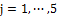denoting the years 2005-2009. The correlations between the inputs and between the observations from different herds and years are no need to consider in the neural network model, which is an advantage of using this model. For an observation, the potential risk factors are the inputs in the Figure 1, and the herd status is the output in the Figure. There are more than 30 explanatory variables and it is unreasonable to put all them in model building. The associations between the response variable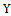and each explanatory variable in a univariate analysis are firstly examined using Spearman’s rank correlation coefficient. Many explanatory variables were skewed and outliers were present and Spearman’s rank correlation was chosen as it is not sensitive to outliers. Explanatory variables are considered for inclusion in the network model if an association significant at the 0.1 level was found from the univariate analysis.

In the neural network model, only one hidden layer with 3 node is used. A weighted sum of the inputs for the ijth observation with p explanation variable for the first unit in the first hidden layer is calculated as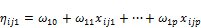(1)

where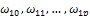are the weights to be estimated by the iterative algorithm to be described later, and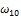is called the bias. The weighted sum of the inputs for the ijth observation for the other two units is similar to the equation (1) but with different weight. The hyperbolic tangent transfer function is applied to weighted sum in each node, and it is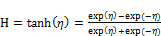(2)

The effect of this transformation is to map values of, which can range from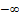to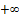, into the narrower range of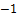to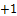. For the computation in the output layer, the algorithm is similar to that in hidden layer, except that only one output node is used here. The weighted linear sum is similar to equation (1), but the explanation variables are changed to be the results of transfer functions, and updated with new weights. Because the target variable is binary and the weighted linear sum combined in this node is range fromto, a logistic activation function is used to output the final result, i.e.,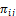, the probability of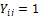.

To estimate the parameters and prevent over-fitting in neural network model fitting, the observations are divided into three part of Training data set (50%), Validation data set (30%), and Test data set (20%). The Training data set is used for preliminary model fitting, and the Validation data set is used for selecting the optimum weights. The weights are estimated iteratively using the training data set in such a way that the error function is minimized. In the case of bTB response data, the following Bernoulli error function is used: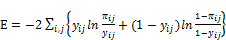(3)

Each iteration yields a set of weights, and each set of weight defines a model. Validation data set are used to choose the models defined by training data. The average squared error are set to be model selection criterion, and the algorithm selects the set of weights that results in the smallest error where the error is calculated from the Validation data set. Since both the Training and Validation data sets are used for parameter estimation and parameter selection, respectively, an additional holdout data set is required for an independent assessment of the model. The Test data set is set aside for this purpose. Models were fitted using the Logistic procedure and Enterprise Miner in SAS version 9.4 (SAS Institute Inc., Cary, NC, USA).

3. Results

From 2005 to 2009, there were 609 distinct herds in the study, giving 2666 observations. Table 1 presents the number of herds and the percentage restricted on an annual basis, and the total herds and percentage restricted for each year keep stable and are around 540 and 4% respectively. In the univariate analysis, herd bTB restriction status was significantly associated with 15 explanatory variables (Table 2). The remaining variables which were not significantly associated herd bTB restriction status are deleted from next model fitting. In the neural network model fitting, the significant variables are included.

Table 1. Number of herds and percentage of these herds with confirmed restrictions for tuberculosis in West Wicklow, Ireland from 2005 to 2009. A herd was considered restricted if any bTB reactor was found on any bTB test in the year.

 Year Total herds Number of restricted herds Percentage restricted* 2005 555 25 0.045 2006 550 22 0.04 2007 530 17 0.032 2008 517 29 0.056 2009 514 29 0.056

*Percentage restricted= Number of herds restricted/ Total number of herds.

Table 2. Spearman’s rank correlation between explanatory variables and herd bTB restriction status (1=restricted, 0=not restricted). The variables with p value<0.1 are listed in Table 2. A1, A2, A3, P1, P2, and P3 were the amplitude and phase of the first, second, and third annual cycle of change related to monthly average temperature and monthly average vapour pressure deficit (VPD) respectively.

 Explanatory variables Spearman’s correlation coefficient P value Herd size 0.14 <.0001 Presence /absence of commonage 0.03 0.08 Total farm area 0.11 <.0001 Total farm perimeter 0.12 <.0001 Herd bTB history 1 0.09 <.0001 Herd bTB history 2 0.09 <.0001 Herd bTB history 3 0.08 <.0001 Herd bTB history of past 3 years 0.12 <.0001 Annual total rainfall 0.05 0.01 Annual max monthly rainfall 0.04 0.03 Annual mean monthly temperature -0.04 0.04 Temperature.A3 0.04 0.04 Annual mean monthly VPD -0.04 0.05 VPD.A2 -0.04 0.03 VPD.P3 0.04 0.05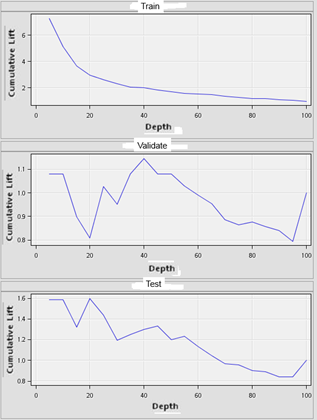Figure 4. The cumulative lift charts of Neural network model fitting results on Training, Validate and Test data set.

The estimation process of model fitting on training data with Bernoulli error function (Equation 3) requires 50 iterations to be convergent. By the model selection procedure in validate data set with average squared error criterion, the weights from the 10th iteration are selected. After 10th iteration, the average squared error starts to increase in the validate data set, although it continued to decline in the training data set. In order to assess the predictive performance of the neural network model, the lift charts for the Training, Validation, and Test data sets are shown in Figure 4. The lift and capture rates calculated from the Test data set are used for evaluating the models or comparing the models because the Test data set is not used in training or fine-tuning the model. To create lift chart, the estimated neural network model are used to calculate the probability of getting herd restricted status for each observation, then the observations are sorted descending by their probability. Then it divides the data set into 20 equal segments called Percentiles. Since the percentiles are created from the sorted data set based on the computed probabilities, the first percentile (called the top percentile) has the customers with the highest mean probability of cancellation. The lift in a given percentile is the actual observed cancellation rate in that percentile divided by the overall actual herd restricted rate.

It can be seen from the Figure 4 that the lift value is highest in the training data set, but worst in validate set. As the model estimation is based on training data, generally the lift on it should perform reasonable. In the first 5% of the observations in the training data set, the herd restricted rate is 32.8358% comparing with 4.5% of overall restricted rate. However, for the Test data set, in the first 5% of observations, the herd restricted rate is 7.4074% comparing with 4.7% of overall restricted rate. Although the fitted neural model does not have high lift value on test data set, it still can be used to enhance work efficiency. For example in a prevention project of herd bTB, based on time and economic consideration, the Irish government may not examine all the herds in the country. Instead, they would random select 5% of herds and detect the bTB incidence. By the neural network model results, they could select 1.58 times herds with bTB reactors more than by random select, which is very useful for the prevention project.

4. Conclusion

This paper use the neural network model to an epidemiological study, i.e. bovine tuberculosis (bTB) occurrence in cattle herds, together with well-established risk factors in the area known as West Wicklow, in the east of Ireland. The binary target variable is whether the herd is in the restricted status, which is defined by whether any bTB reactor is detected in the herd. To estimate the parameters and prevent over-fitting in neural network model fitting, the observations are divided into three part of Training data set (50%), Validation data set (30%), and Test data set (20%). The Training data set is used for preliminary model fitting, and the Validation data set is used for selecting the optimum weights. The weights are estimated iteratively using the training data set in such a way that the error function is minimized. Although the fitted neural model does not have high lift value on test data set, it still can be used to enhance work efficiency. For example in a prevention project of herd bTB with only 5% selection of total herds, the fitted neural network model could select 1.58 times herds with bTB reactors more than by random select, which is very useful for the prevention project.

Acknowledgements

The first author wish to thank to DAFM for providing all the herd data through CVERA, University College Dublin, and Met Éireann for providing weather data. This paper is funded by the project of National Natural Science Fund, Logistics distribution of artificial order picking random process model analysis and research (Project number: 71371033); and funded by intelligent logistics system Beijing Key Laboratory (No.BZ0211); and funded by scientific-research bases---Science & Technology Innovation Platform---Modern logistics information and control technology research (Project number: PXM2015_014214_000001); University Cultivation Fund Project of 2014-Research on Congestion Model and algorithm of picking system in distribution center (0541502703).

References

1. Afifi, A.A and Clark, Virginia, Computer Aided Multivariate Analysis, CRC Press, 2004.
2. Bishop, C.M. (1995) Neural Networks for Pattern Recognition. New York: Oxford University Press.
3. Biondo S., Ramos E., Deiros M. et al. Prognostic factors for mortality in left colonic peritonitis: a new scoring system // J. Am. Coll. Surg. – 2000. – Vol. 191, No. 6. – Р. 635-642.
4. Boyd, C. R.; Tolson, M. A.; Copes, W. S. (1987). "Evaluating trauma care: The TRISS method. Trauma Score and the Injury Severity Score". The Journal of trauma 27 (4): 370–378.
5. Collett, D., 2002, Modelling binary data. Chapman & Hall/CRC, London, 129-213 pp.
6. David A. Freedman (2009). Statistical Models: Theory and Practice.Cambridge University Press. p. 128.
7. Gareth James; Daniela Witten; Trevor Hastie; Robert Tibshirani (2013).An Introduction to Statistical Learning. Springer. p. 6.
8. Gordejo, R.F.J., Vermeersch, J.P., 2006. Towards eradication of bovine tuberculosis in the European Union. European Union Veterinary Microbiology 112, 101-109.
9. Griffin, J.M.,Hahesya, T.,Lyncha, T.,M.D. Salmanb, M.D.,McCarthya, J.,Hurleya, T., 1993. The association of cattle husbandry practices, environmental factors and farmer characteristics with the occurence of chronic bovine tuberculosis in dairy herds in the Republic of Ireland.Preventive Veterinary Medicine17, 145-160.
10. Griffin, J.M., Williams, D.H., Kelly, G.E., Clegg, T.A., O’Boyle, I., Collins, J.D., More, S.J., 2005. The impact of badger removal on the control of tuberculosis in cattle herds in Ireland. Preventive Veterinary Medicine 67, 237–266.
11. Hahesy, T., Kelleher, D.L., Doherty, J., 1992. An investigation of a possible association between the occurrence of bovine tuberculosis and weather variables. Irish Veterinary Journal 45, 127-128.
12. Kattamuri S. Sarma (2013). Predictive Modeling with SAS Enterprise Miner Practical Solutions for Business Applications Second Edition. NC: SAS Institute Inc, Cary.
13. Kologlu M., Elker D., Altun H., Sayek I. (2001) Valdation of MPI and OIA II in two different groups of patients with secondary peritonitis // Hepato-Gastroenterology. – 2001. – Vol. 48, No. 37. – P. 147-151.
14. Manro, S. and Kumam, P.(2012) Common fixed point theorems for expansion mappings in various abstract spaces using the concept of weak reciprocal continuity, Fixed Point Theory and Applications, 2012:221.
15. Ma, E., Lam, T., Wong, C., Chuang, S.K., 2010. Is hand, foot and mouth disease associated with meteorological parameters?. Epidemiology and Infection 138, 1779-1788.
16. SAS Institute Inc, 2013. SAS/STAT® 9.4 User’s Guide: The GLIMMIX Procedure (Book Excerpt). NC: SAS Institute Inc, Cary.
17. SAS Institute Inc, 2013. SAS/STAT® 9.4 User’s Guide: The Logistic Procedure (Book Excerpt). NC: SAS Institute Inc, Cary.
18. Walker, SH; Duncan, DB (1967). "Estimation of the probability of an event as a function of several independent variables".Biometrika 54: 167–178.

 Contents 1. 2. 3. 4.
Article ToolsAbstractPDF(581K)